Function Repository Resource:

# EvoluteCurve

Compute the evolute of a curve

Contributed by: Wolfram Staff (original content by Alfred Gray)
 ResourceFunction["EvoluteCurve"][c,t] computes the evolute of the curve c.

## Details

An evolute is the locus of the centers of curvature of a plane curve, or the envelope of a plane curve's normals.

## Examples

### Basic Examples (3)

Define the curve of an ellipse:

 In:=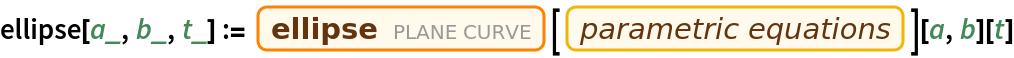Compute its evolute:

 In:=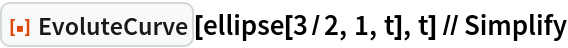Out=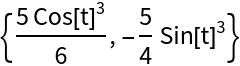Plot the ellipse and evolute:

 In:=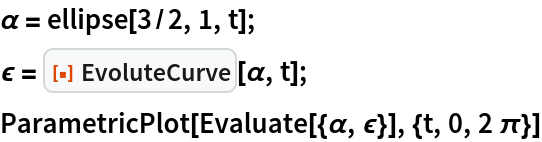Out=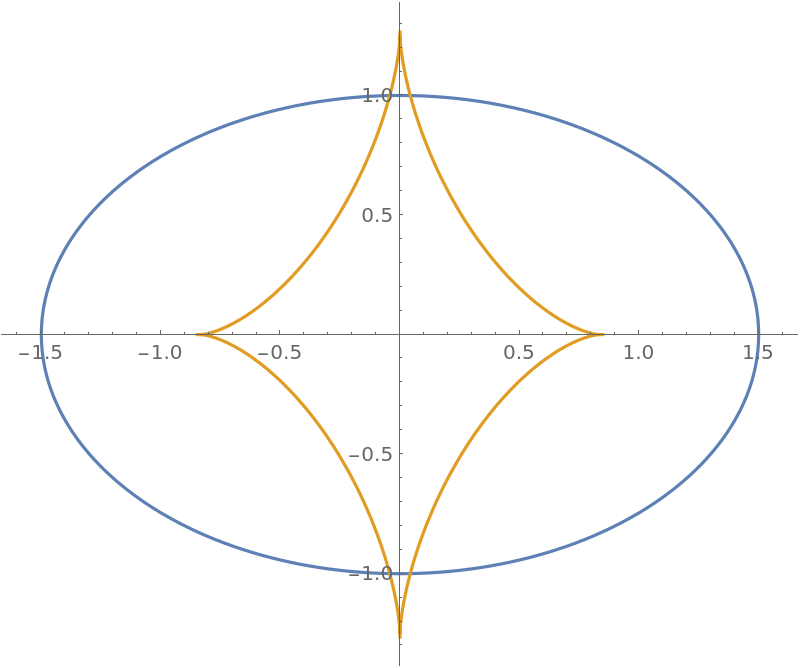Define a curve known as a tractrix:

 In:=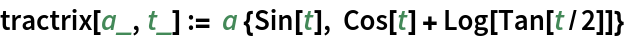Its evolute is a catenary:

 In:=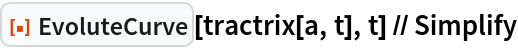Out=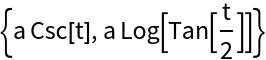Plot the result:

 In:=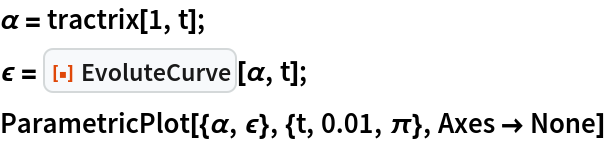Out=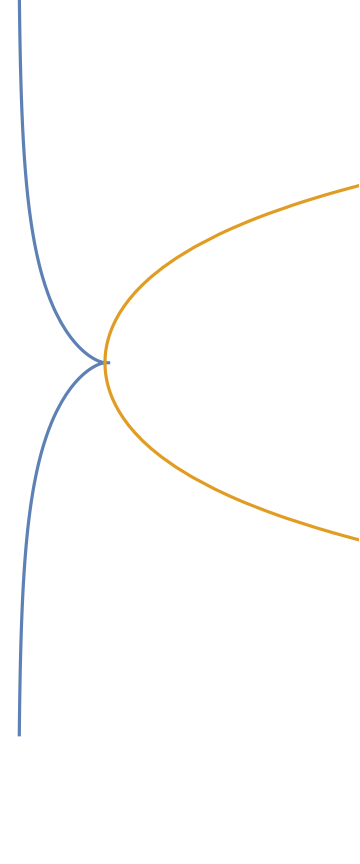Define a curve called a cissoid:

 In:=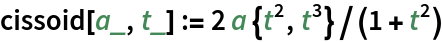Plot repeated evolutes of the curve:

 In:=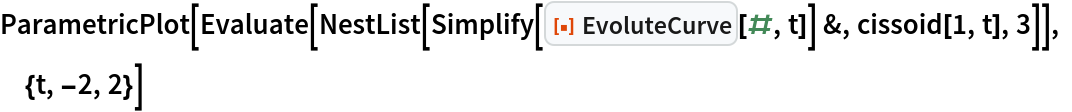Out=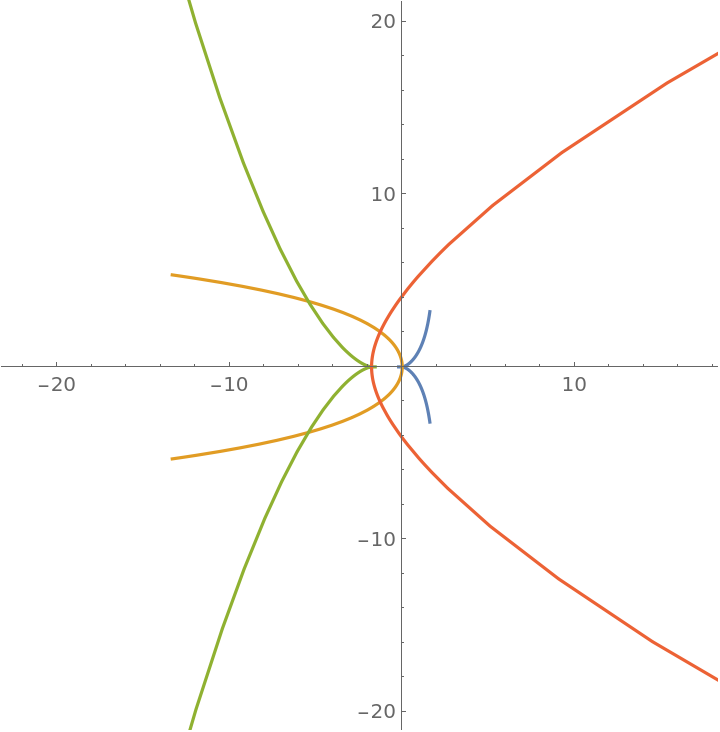### Applications (2)

Evolute of a cycloid:

 In:=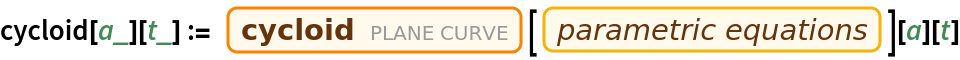In:=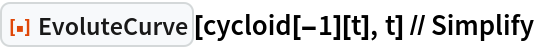Out=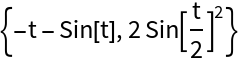A cycloidal pendulum, which exhibits the tautochrone property:

 In:=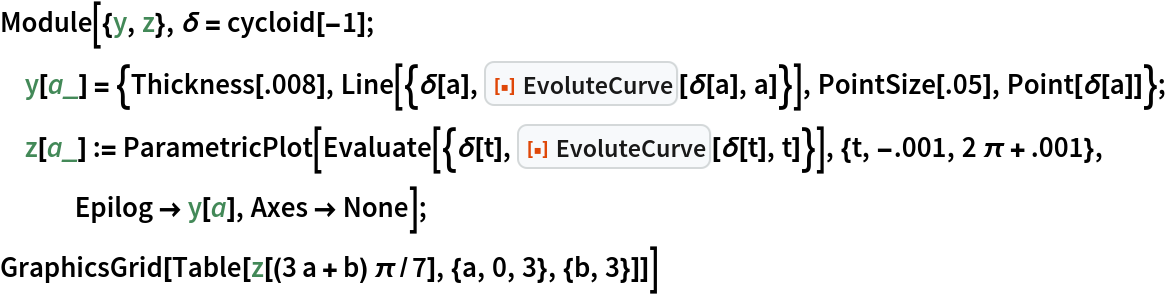Out=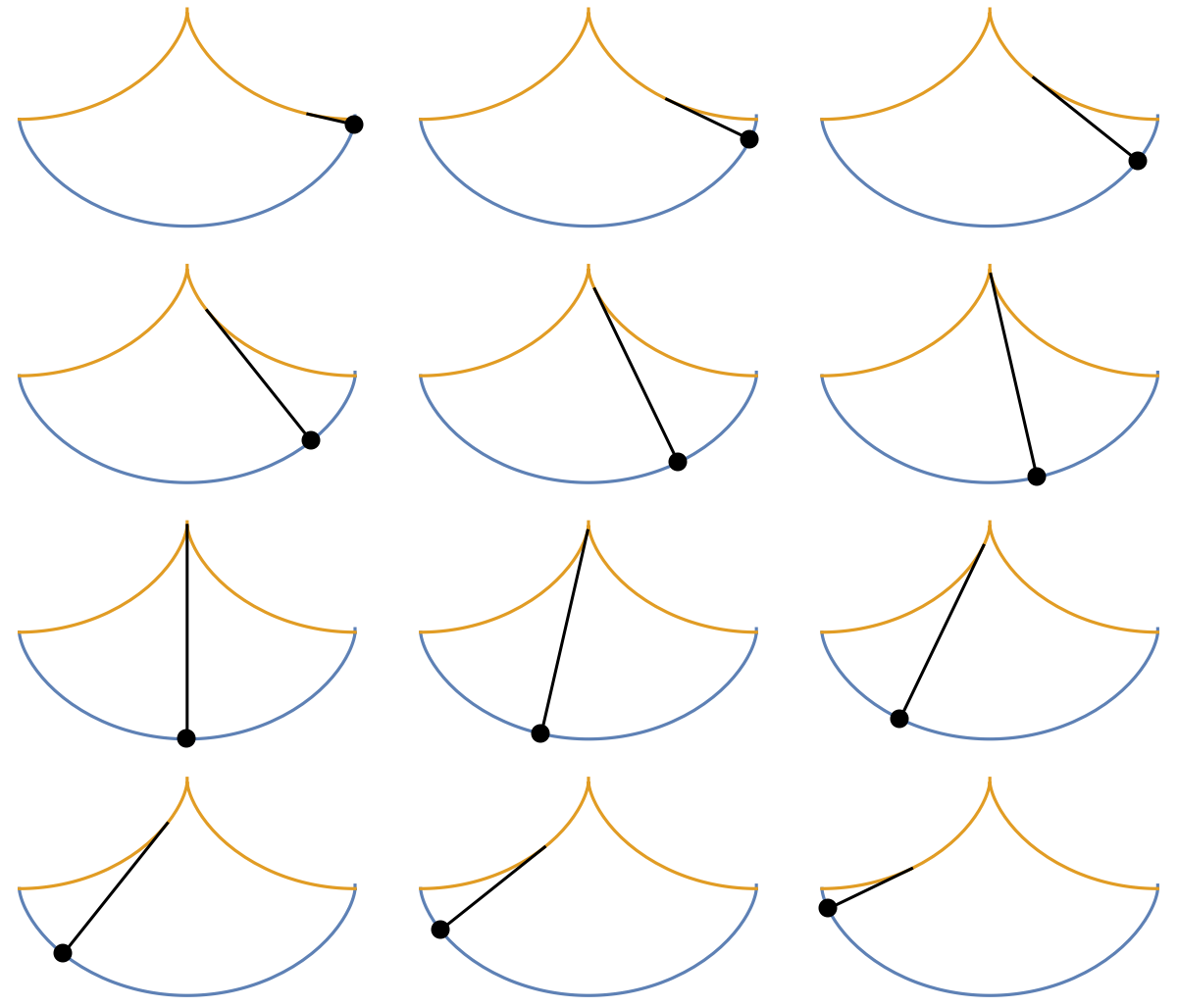### Properties and Relations (1)

The evolute of a curve can be expressed in terms of the curvature and the normal vector:

 In:=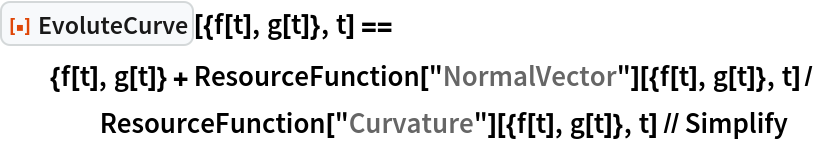Out=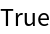### Neat Examples (1)

Show the evolute as an envelope of normals:

 In:=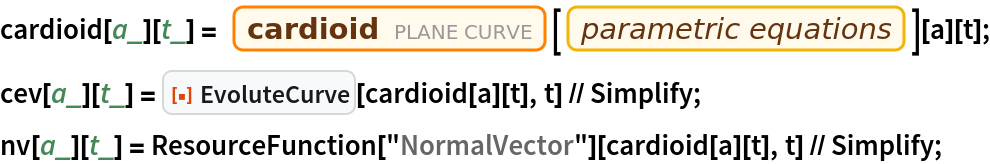In:=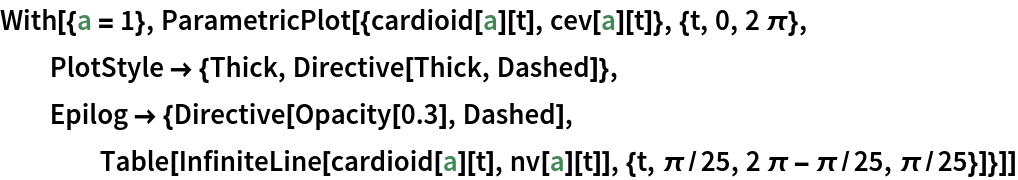Out=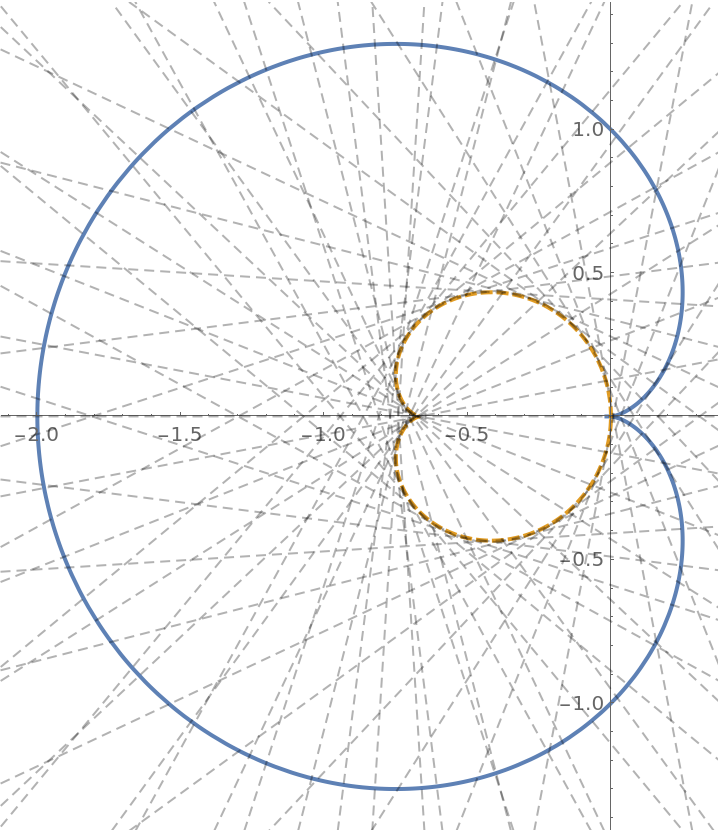Enrique Zeleny

## Version History

• 1.0.1 – 08 March 2021
• 1.0.0 – 03 March 2020